# Vectors

One of the first models learned in physics are the equations governing the laws of motion with constant acceleration: $x(t) = x_0 + v_0 t + 1/2 \cdot a t^2$. This is a consequence of Newton's second law of motion applied to the constant acceleration case. A related formula for the velocity is $v(t) = v_0 + at$. The following figure is produced using these formulas applied to both the vertical position and the horizontal position: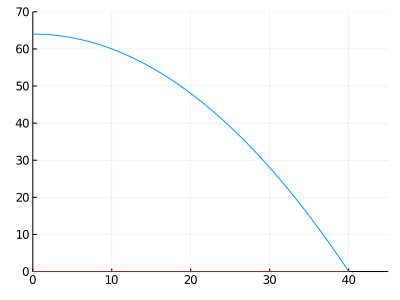Position, velocity, and acceleration vectors (scaled) for projectile motion. Vectors are drawn with tail on the projectile. The position vector (black) points from the origin to the projectile, the velocity vector (red) is in the direction of the trajectory, and the acceleration vector (green) is a constant pointing downward.

For the motion in the above figure, the object's $x$ and $y$ values change according to the same rule, but, as the acceleration is different in each direction, we get different formula, namely: $x(t) = x_0 + v_{0x} t$ and $y(t) = y_0 + v_{0y}t - 1/2 \cdot gt^2$.

It is common to work with both formulas at once. Mathematically, when graphing, we naturally pair off two values using Cartesian coordinates (e.g., $(x,y)$). Another means of combining related values is to use a vector. The notation for a vector varies, but to distinguish them from a point we will use $\langle x,~ y\rangle$. With this notation, we can use it to represent the position, the velocity, and the acceleration at time $t$ through:

~ \begin{align} \vec{x} &= \langle x_0 + v_{0x}t,~ -(1/2) g t^2 + v_{0y}t + y_0 \rangle,\\ \vec{v} &= \langle v_{0x},~ -gt + v_{0y} \rangle, \text{ and }\\ \vec{a} &= \langle 0,~ -g \rangle. \end{align} ~

Don't spend time thinking about the formulas if they are unfamiliar. The point emphasized here is that we have used the notation $\langle x,~ y \rangle$ to collect the two values into a single object, which we indicate through a label on the variable name. These are vectors, and we shall see they find use far beyond this application.

Initially, our primary use of vectors will be as containers, but it is worthwhile to spend some time to discuss properties of vectors and their visualization.

A line segment in the plane connects two points $(x_0, y_0)$ and $(x_1, y_1)$. The length of a line segment (its magnitude) is given by the distance formula $\sqrt{(x_1 - x_0)^2 + (y_1 - y_0)^2}$. A line segment can be given a direction by assigning an initial point and a terminal point. A directed line segment has both a direction and a magnitude. A vector is an abstraction where just these two properties

• a direction and a magnitude - are intrinsic. While a directed line

segment can be represented by a vector, a single vector describes all such line segments found by translation. That is, how the the vector is located when visualized is for convenience, it is not a characteristic of the vector. In the figure above, all vectors are drawn with their tails at the position of the projectile over time.

We can visualize a (two-dimensional) vector as an arrow in space. This arrow has two components. We represent a vector than mathematically as $\langle x,~ y \rangle$. For example, the vector connecting the point $(x_0, y_0)$ to $(x_1, y_1)$ is $\langle x_1 - x_0,~ y_1 - y_0 \rangle$.

The magnitude of a vector comes from the distance formula applied to a line segment, and is $\| \vec{v} \| = \sqrt{x^2 + y^2}$.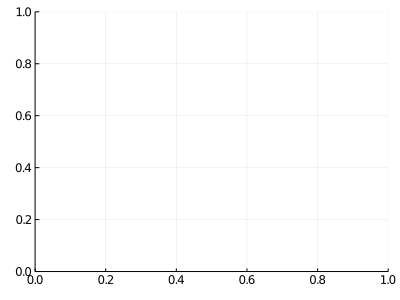A vector and its unit vector. They share the same direction, but the unit vector has a standardized magnitude.

We call the values $x$ and $y$ of the vector $\vec{v} = \langle x,~ y \rangle$ the components of the $v$.

Two operations on vectors are fundamental.

• Vectors can be multiplied by a scalar (a real number): $c\vec{v} = \langle cx,~ cy \rangle$. Geometrically this scales the vector by a factor of $\lvert c \rvert$ and switches the direction of the vector by 180 degree when $c < 0$. A unit vector is one with magnitude 1, and, except for the $\vec{0}$ vector, can be formed from $\vec{v}$ by dividing $\vec{v}$ by its magnitude. A vector's two parts are summarized by its direction given by a unit vector gives and its norm given by the magnitude.

• Vectors can be added: $\vec{v} + \vec{w} = \langle v_x + w_x,~ v_y + w_y \rangle$. That is, each corresponding component adds to form a new vector. Similarly for subtraction. The $\vec{0}$ vector then would be just $\langle 0,~ 0 \rangle$ and would satisfy $\vec{0} + \vec{v} = \vec{v}$ for any vector $\vec{v}$. The vector addition $\vec{v} + \vec{w}$ is visualized by placing the tail of $\vec{w}$ at the tip of $\vec{v}$ and then considering the new vector with tail coming from $\vec{v}$ and tip coming from the position of the tip of $\vec{w}$. Subtraction is different, place both the tails of $\vec{v}$ and $\vec{w}$ at the same place and the new vector has tail at the tip of $\vec{v}$ and tip at the tip of $\vec{w}$.The sum of two vectors can be visualized by placing the tail of one at the tip of the otherThe sum of two vectors can be visualized by placing the tail of one at the tip of the other

The concept of scalar multiplication and addition, allow the decomposition of vectors into standard vectors. The standard unit vectors in two dimensions are $e_x = \langle 1,~ 0 \rangle$ and $e_y = \langle 0,~ 1 \rangle$. Any two dimensional vector can be written uniquely as $a e_x + b e_y$ for some pair of scalars $a$ and $b$ (or as, $\langle a, b \rangle$). This is true more generally where the two vectors are not the standard unit vectors - they can be any two non-parallel vectors.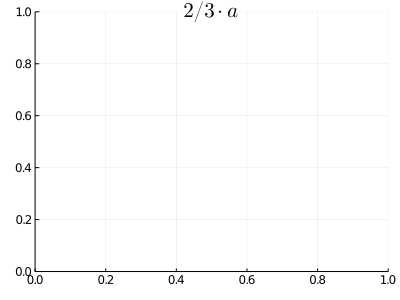The vector $\langle 4,3 \rangle$ is written as $2$/$3$ $\cdot\langle 1,2 \rangle$ $+$ $5$/$3$ $\cdot\langle 2,1 \rangle$. Any vector $\vec{c}$ can be written uniquely as $\alpha\cdot\vec{a} + \beta \cdot \vec{b}$ provided $\vec{a}$ and $\vec{b}$ are not parallel.

The two operations of scalar multiplication and vector addition are defined in a component-by-component basis. We will see that there are many other circumstances where performing the same action on each component in a vector is desirable.

When a vector is placed with its tail at the origin, it can be described in terms of the angle it makes with the $x$ axis, $\theta$, and its length, $r$. The following formulas apply:

$~ r = \sqrt{x^2 + y^2}, \quad \tan(\theta) = y/x. ~$

If we are given $r$ and $\theta$, then the vector is $v = \langle r \cdot \cos(\theta),~ r \cdot \sin(\theta) \rangle$.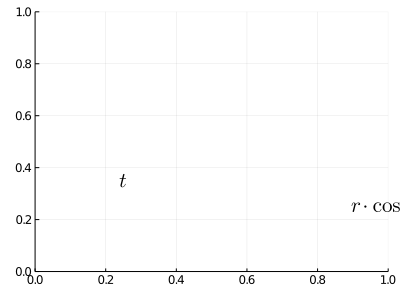A vector $\langle x, y \rangle$ can be written as $\langle r\cdot \cos(\theta), r\cdot\sin(\theta) \rangle$ for values $r$ and $\theta$. The value $r$ is a magnitude, the direction parameterized by $\theta$.

## Vectors in Julia

A vector in Julia can be represented by its individual components, but it is more convenient to combine them into a collection using the [,] notation:

x, y = 1, 2
v = [x, y]        # square brackets, not angles

2-element Array{Int64,1}:
1
2


The basic vector operations are implemented for vector objects. For example, the vector v has scalar multiplication defined for it:

10 * v

2-element Array{Int64,1}:
10
20


The norm function returns the magnitude of the vector (by default):

import LinearAlgebra: norm
norm(v)

2.23606797749979


A unit vector is then found by scaling by the reciprocal of the magnitude:

v / norm(v)

2-element Array{Float64,1}:
0.4472135954999579
0.8944271909999159


In addition, if w is another vector, we can add and subtract:

w = [3, 2]
v + w, v - 2w

([4, 4], [-5, -2])


We see above that scalar multiplication, addition, and subtraction can be done without new notation. This is because the usual operators have methods defined for vectors.

Finally, to find an angle $\theta$ from a vector $\langle x,~ y\rangle$, we can employ the atan function using two arguments:

# v  = [x, y]
norm(v), atan(y, x)

(2.23606797749979, 1.1071487177940904)


## Higher dimensional vectors

Mathematically, vectors can be generalized to more than 2 dimensions. For example, using 3-dimensional vectors are common when modeling events happening in space, and 4-dimensional vectors are common when modeling space and time.

In Julia there are many uses for vectors outside of physics applications. A vector in Julia is just a one-dimensional collection of similarly typed values. Such objects find widespread usage. For example:

• In plotting graphs with Julia, vectors are used to hold the $x$ and $y$ coordinates of a collection of points to plot and connect with straight lines. There can be hundreds of such points in a plot.

• Vectors are a natural container to hold the roots of a polynomial or zeros of a function.

• Vectors may be used to record the state of an iterative process.

• Vectors are naturally used to represent a data set, such as arise when collecting survey data.

Creating higher-dimensional vectors is similar to creating a two-dimensional vector, we just include more components:

fibs = [1,1,2,3,5,8,13]

7-element Array{Int64,1}:
1
1
2
3
5
8
13


Later we will discuss different ways to modify the values of a vector to create new ones, similar to how scalar multiplication does.

As mentioned, vectors in Julia are comprised of elements of a similar type, but the type is not limited to numeric values. For example, a vector of strings might be useful for text processing, a vector of Boolean values can naturally arise, some applications are even naturally represented in terms of vectors of vectors. Look at the output of these two vectors:

["one", "two", "three"]  # Array{T, 1} is shorthand for Vector{T}. Here T - the type - is String

3-element Array{String,1}:
"one"
"two"
"three"

[true, false, true]		# vector of Bool values

3-element Array{Bool,1}:
1
0
1


Finally, we mention that if Julia has values of different types it will promote them to a common type if possible. Here we combine three types of numbers, and see that each is promoted to Float64:

[1, 2.0, 3//1]

3-element Array{Float64,1}:
1.0
2.0
3.0


Whereas, in this example where there is no common type to promote the values to, a catch-all type of Any is used to hold the components.

["one", 2, 3.0, 4//1]

4-element Array{Any,1}:
"one"
2
3.0
4//1


## Indexing

Getting the components out of a vector can be done in a manner similar to multiple assignment:

v = [1, 2]
x, y = v

2-element Array{Int64,1}:
1
2


When the same number of variable names are on the left hand side of the assignment as in the container on the right, each is assigned in order.

Though this is convenient for small vectors, it is far from being so if the vector has a large number of components. However, the vector is stored in order with a first, second, third, $\dots$ component. Julia allows these values to be referred to by index. This too uses the [] notation, though differently. Here is how we get the second component of v:

v

2


The last value of a vector is usually denoted by $v_n$. In Julia, the length function will return $n$, the number of items in the container. So v[length(v)] will refer to the last component. However, the special keyword end will do so as well, when put into the context of indexing. So v[end] is more idiomatic.

### Assignment and indexing

This notation can also be used for assignment. The following expression replaces the second component with a new value:

v = 10

10


The right hand side is returned, not the value for v. We can check that v is now $\langle 1,~ 10 \rangle$ by showing it:

v

2-element Array{Int64,1}:
1
10


The assignment v is different than the initial assignment v=[1,2] in that, v=10 modifies the container that v points to, whereas v=[1,2] replaces the binding for v. The indexed assignment is then more memory efficient when vectors are large. This point is also of interest when passing vectors to functions, as a function may modify components of the vector passed to it, though can't replace the container itself.

## Some functions useful when working with vectors.

As mentioned, the length function returns the number of components in a vector. It is one of several useful functions for vectors.

The sum and prod function will add and multiply the elements in a vector:

v = [1,1,2,3,5,8]
sum(v), prod(v)

(20, 240)


The unique function will throw out any duplicates:

unique(v) # drop a 1

5-element Array{Int64,1}:
1
2
3
5
8


The functions maximum and minimum will return the largest and smallest values of an appropriate vector.

v = [1,4,2,3]
maximum(v)

4


(These should not be confused with max and min which give the largest or smallest value over all their arguments.)

The extrema function returns both the smallest and largest value of a collection:

extrema(v)

(1, 4)


The sort function will rearrange the values in v:

sort(v)

4-element Array{Int64,1}:
1
2
3
4


The keyword argument, rev=false can be given to get values in decreasing order:

sort(v, rev=false)

4-element Array{Int64,1}:
1
2
3
4


For adding a new element to a vector the push! method can be used, as in

push!(v, 5)

5-element Array{Int64,1}:
1
4
2
3
5


To append more than one value, the append! function can be used:

append!(v, [6,8,7])

8-element Array{Int64,1}:
1
4
2
3
5
6
8
7


These two functions modify or mutate the values stored within the vector v that passed as an argument. In the push! example above, the value 5 is added to the vector of 4 elements. In Julia, a convention is to name mutating functions with a trailing exclamation mark. (Again, these do not mutate the binding of v to the container, but do mutate the contents of the container.) There are functions with mutating and non-mutating definitions, an example is sort and sort!.

If only a mutating function is available, like push!, and this is not desired a copy of the vector can be made. It is not enough to copy by assignment, as with w = v. As both w and v will be bound to the same memory location. Rather, you call copy to make a new container with copied contents, as in w = copy(v).

Creating new vectors of a given size is common for programming, though not much use will be made here. There are many different functions to do so: ones to make a vector of ones, zeros to make a vector of zeros, trues and falses to make Boolean vectors of a given size, and similar to make a similar-sized vector (with no particular values assigned).

## Applying functions element by element to values in a vector

Functions such as sum or length are known as reductions as they reduce the "dimensionality" of the data: a vector is in some sense $1$-dimensional, the sum or length $0$-dimensional. Applying a reduction is straightforward, it is just a regular function call.

Other desired operations with vectors act differently. Rather than reduce a collection of values using some formula, the goal is to apply some formula to each of the values, returning a modified vector. A simple example might be to square each element, or subtract the average value from each element. An example comes from statistics. When computing a variance, we start with data $x_1, x_2, \dots, x_n$ and along the way form the values $(x_1-\bar{x})^2, (x_2-\bar{x})^2, \dots, (x_n-\bar{x})^2$.

Such things can be done in many differents ways. Here we describe two, but will primarily utilize the first.

### Broadcasting a function call

If we have a vector, xs, and a function, f, to apply to each value, there is a simple means to achieve this task. By adding a "dot" between the function name and the parenthesis that enclose the arguments, instructs Julia to "broadcast" the function call. The details allow for more flexibility, for this purpose, broadcasting will take each value in xs and apply f to it, returning a vector of the same size as xs. When more than one argument is involved, broadcasting will try to fill out different sized objects.

For example, the following will find, using sqrt, the square root each value in a vector:

xs = [1, 1, 3, 4, 7]
sqrt.(xs)

5-element Array{Float64,1}:
1.0
1.0
1.7320508075688772
2.0
2.6457513110645907


This would find the sine of each number in xs:

sin.(xs)

5-element Array{Float64,1}:
0.8414709848078965
0.8414709848078965
0.1411200080598672
-0.7568024953079282
0.6569865987187891


The ^ operator is an infix operator. It too can be broadcast by using the form .^, as in:

xs .^ 2

5-element Array{Int64,1}:
1
1
9
16
49


Here is an example involving the logarithm of a set of numbers. In astronomy, a logarithm with base $100^{1/5}$ is used for star brightness. We can use broadcasting to find this value for several values at once through:

xs = [1/5000, 1/500, 1/50, 1/5, 5, 50]
b = (100)^(1/5)
log.(b, xs)

6-element Array{Float64,1}:
-9.247425010840049
-6.747425010840047
-4.247425010840047
-1.747425010840047
1.747425010840047
4.247425010840047


Broadcasting with multiple arguments allows for mixing of vectors and scalar values, as above, making it convenient when parameters are used.

As a final example, the task from statistics of centering and then squaring can be done with broadcasting. We go a bit further, showing how to compute the (unbiased) sample variance of a data set. This has the formula $(1/(n-1))\cdot ((x_1-\bar{x})^2 + \cdots + (x_n + \bar{x})^2)$. It can be computed, with broadcasting, through:

import Statistics: mean
xs = [1, 1, 2, 3, 5, 8, 13]
n = length(xs)
(1/(n-1)) * sum(abs2.(xs .- mean(xs)))

19.57142857142857


This shows many of the manipulations that can be made with vectors. Rather than write .^2, we follow the defintion of var and chose the possibly more performant abs2 function which, in general, efficiently finds $|x|^2$ for various number types. The .- uses broadcasting to subtract a scalar (mean(xs)) from a vector (xs). Without the ., this would error.

### Comprehensions

In mathematics, set notation is often used to describe elements in a set.

For example, the first 5 cubed numbers can be described by:

$~ \{x^3: x \text{ in } 1, 2,\dots, 5\} ~$

Comprehension notation is similar. The above could be created in Julia with:

xs = [1,2,3,4,5]
[x^3 for x in xs ]

5-element Array{Int64,1}:
1
8
27
64
125


Something similar can be done more succinctly:

xs .^ 3

5-element Array{Int64,1}:
1
8
27
64
125


However, comprehensions have a value when more complicated expressions are desired as they work with an expression of x, and not a pre-defined or user-defined function.

Another typical example of set notation might include a condition, such as, the numbers divisible by $7$ between $1$ and $100$. Set notation might be:

$~ \{x: rem(x, 7) = 0 \text{ for } x \text{ in } 1, 2, \dots, 100\}. ~$

This would be read: "the set of $x$ such that the remainder on division by $7$ is $0$ for all x in $1, 2, \dots, 100$."

In Julia, a comprehension can include an if clause to mirror, somewhat, the math notation. For example, the above would become (using 1:100 as a means to create the numbers $1,2,\dots, 100$, as will be described in a later section):

[x for x in 1:100 if rem(x,7) == 0]

14-element Array{Int64,1}:
7
14
21
28
35
42
49
56
63
70
77
84
91
98


Comprehensions can be a convenient means to describe a collection of numbers, especially when no function is defined, but the simplicity of the broadcast notation (just adding a judicious ".") leads to its more common use in these notes.

##### Example: creating a "T" table for creating a graph

The process of plotting a function is usually first taught by generating a "T" table: values of $x$ and corresponding values of $y$. These pairs are then plotted on a Cartesian grid and the points are connected with lines to form the graph. Generating a "T" table in Julia is easy: create the $x$ values, then create the $y$ values for each $x$.

To be concrete, let's generate $7$ points to plot $f(x) = x^2$ over $[-1,1]$.

The first task is to create the xs. We will see later, more convenient ways to generate patterned data, but for now, we do this by hand:

a,b, n = -1, 1, 7
d = (b-a) // (n-1)
xs = [a, a+d, a+2d, a+3d, a+4d, a+5d, a+6d]  # 7 points

7-element Array{Rational{Int64},1}:
-1//1
-2//3
-1//3
0//1
1//3
2//3
1//1


To get the corresponding $y$ values, we can use a compression (or define a function and broadcast):

ys = [x^2 for x in xs]

7-element Array{Rational{Int64},1}:
1//1
4//9
1//9
0//1
1//9
4//9
1//1


Vectors can be compared together by combining them into a separate container, as follows:

[xs ys]

7×2 Array{Rational{Int64},2}:
-1//1  1//1
-2//3  4//9
-1//3  1//9
0//1  0//1
1//3  1//9
2//3  4//9
1//1  1//1


(If there is a space between objects they are horizontally combined. In our construction of vectors using [] we used a comma for vertical combination. More generally we should use a ; for vertical concatenation.)

In the sequel, we will typically use broadcasting for this task using two steps: one to define a function the second to broadcast it.

## Other container types

Vectors in Julia are a container, one of many different types. Another useful type for programming purposes are tuples. If a vector if formed by placing comma-separated values within a [] pair (e.g., [1,2,3]), a tuple is formed by placing comma-separated values withing a () pair. I tuple of length $1$ uses a convention of a trailing comma to distinguish it from a parethesized expression (e.g. (1,) is a tuple, (1) is just the value 1).

Tuples are used in programming, as they don't typically require memory to be used so they can be faster. Internal usages are for function arguments and function return types. Unlike vectors, tuples can be heterogeneous collections. (When commas are used to combine more than one output into a cell, a tuple is being used.)

Also unlike vectors, tuples can have names which can be used for referening a value, similar to indexing but possibly more convenient. Named tuples as similar to dictionaries which are used to associate a key (like a name) with a value.

## Questions

###### Question

Which command will create the vector $\vec{v} = \langle 4,~ 3 \rangle$?

###### Question

Which command will create the vector with components "4,3,2,1"?

###### Question

What is the magnitude of the vector $\vec{v} = \langle 10,~ 15 \rangle$?

###### Question

Which of the following is the unit vector in the direction of $\vec{v} = \langle 3,~ 4 \rangle$?

###### Question

What vector is in the same direction as $\vec{v} = \langle 3,~ 4 \rangle$ but is 10 times as long?

###### Question

If $\vec{v} = \langle 3,~ 4 \rangle$ and $\vec{w} = \langle 1,~ 2 \rangle$ find $2\vec{v} + 5 \vec{w}$.

###### Question

Let v be defined by:

v = [1, 1, 2, 3, 5, 8, 13, 21]


What is the length of v?

What is the sum of v?

What is the prod of v?

###### Question

From transum.org.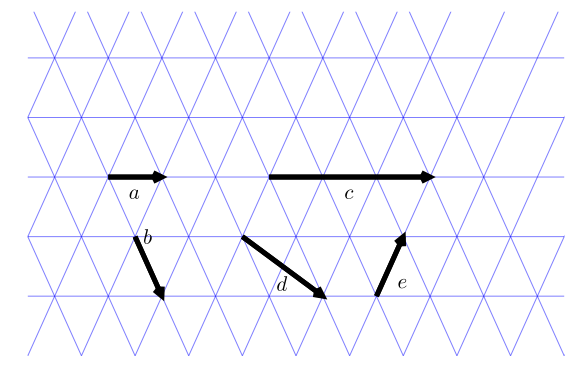The figure shows $5$ vectors.

Express vector c in terms of a and b:

Express vector d in terms of a and b:

Express vector e in terms of a and b:

###### Question

If xs=[1, 2, 3, 4] and f(x) = x^2 which of these will not produce the vector [1, 4, 9, 16]?

###### Question

Let $f(x) = \sin(x)$ and $g(x) = \cos(x)$. In the interval $[0, 2\pi]$ the zeros of $g(x)$ are given by

zs = [pi/2, 3pi/2]

2-element Array{Float64,1}:
1.5707963267948966
4.71238898038469


What construct will give the function values of $f$ at the zeros of $g$?

###### Question

If zs = [1,4,9,16] which of these commands will return [1.0, 2.0, 3.0, 4.0]?